# Colleges with the highest SAT scores in North Dakota

Top 5 colleges in North Dakota with the highest SAT scores
Looking for the colleges with the highest SAT scores in North Dakota? Well you're in luck! We've compiled a national college database and have created a list of the top 5 universities with the highest SAT scores in North Dakota. These are the schools whose applicants had the highest average SAT scores in North Dakota. And, since these tests are meant to determine academic prowess, they are arguably the schools with the most academically proficient students. You could even say these are the best colleges in North Dakota. We also include each college's ACT scores and acceptance rate so that you can see where you would have the easiest or hardest time getting in. Read on to find out more.

## North Dakota State University SAT scores

The average SAT score for North Dakota State University is 1170.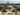The average SAT score of 1170 breaks down into:

• SAT math: 590

The average ACT score for North Dakota State University is 24 and their acceptance rate is 91.6%.

## University of North Dakota SAT scores

The average SAT score for University of North Dakota is 1110.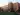The average SAT score of 1110 breaks down into:

• SAT math: 570

The average ACT score for University of North Dakota is 23 and their acceptance rate is 82.2%.

## Mayville State University SAT scores

The average SAT score for Mayville State University is 1040.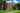The average SAT score of 1040 breaks down into:

• SAT math: 520

The average ACT score for Mayville State University is 20 and their acceptance rate is 57.1%.

## Minot State University SAT scores

The average SAT score for Minot State University is 1030.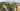The average SAT score of 1030 breaks down into:

• SAT math: 520

The average ACT score for Minot State University is 22 and their acceptance rate is 50.1%.

## Valley City State University SAT scores

The average SAT score for Valley City State University is 1024.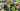The average SAT score of 1024 breaks down into:

• SAT math: 520